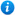# Mixed Numbers and Improper Fractions - Year 5

KS2 Maths Teaching Resources: Mixed Numbers and Improper Fractions - Year 5

71 slide PowerPoint presentation and 9 accompanying worksheets.

Year 5 maths programme of study - Number - fractions (including decimals and percentages):

• recognise mixed numbers and improper fractions and convert from one form to the other and write mathematical statements > 1 as a mixed number [for example,+== 1]

In this maths teaching resource pupils are introduced to mixed numbers and improper fractions and practise converting from one to the other. 'Mixed Numbers and Improper Fractions - Year 5' is an ideal teaching aid to use when teaching the above year 5 curriculum learning objective. Content includes:

• What are fractions recap
• What are proper and improper fractions explanation
• Name the improper fractions activity and worksheet
• What are mixed numbers explanation
• Name the mixed numbers activity and worksheet
• Colour the diagrams to represent the improper fractions and then name the mixed number activity and worksheet
• How to convert an improper fraction into a mixed number explanation
• Converting improper fractions into mixed numbers activity and worksheet x2
• How to convert mixed numbers into improper fractions explanation
• Converting mixed numbers into improper fractions activity and worksheet x2
• Adding fractions with the same denominator and then converting the answer into a mixed number activity and worksheet
• Improper fractions and mixed numbers word problems activity and worksheet
• Improper fractions and mixed numbers interactive class activity
'Mixed Numbers and Improper Fractions - Year 5' is fully editable so teachers can adapt the resource to suit the needs of each class they teach.

To look at 'Mixed Numbers and Improper Fractions - Year 5' in more detail please click on the PowerPoint images above.

Our Price : £3.99 / 3 Credits